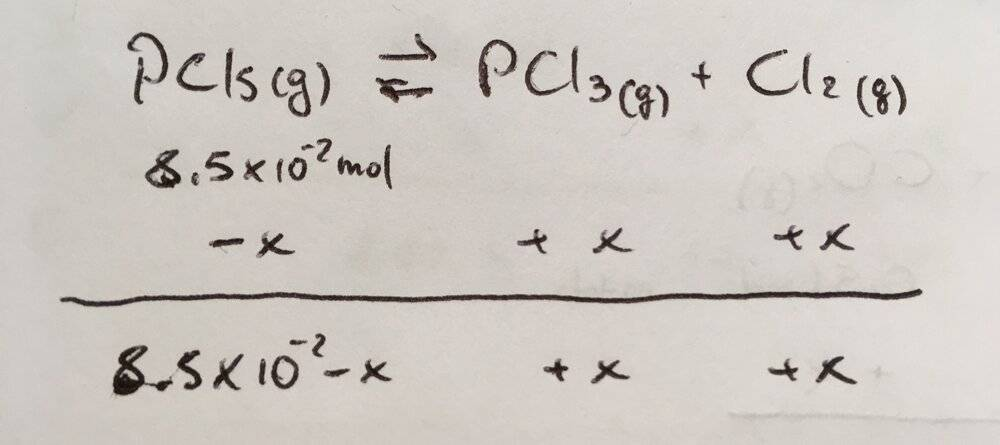# Kp of a gaseous reaction

• Chemistry
• DottZakapa

#### DottZakapa

Homework Statement
##8,5*10^-2## moles of gaseous ##PCL_5## are inserted in a 0,50 L volume reactor. At 540 K, the equilibrium ##PCL_5 <->PCL_3+CL_2## , is achieved and the total pressure in the reactor is 11.10 atm. Calculate Kp at equilibrium at this temperature.
Relevant Equations
Equilibrium
I am stuck, i have compute the total moles as :
## n_{tot}=\frac{P_{tot*V}}{RT}##=0,125 mol

##Kp= \frac{P_{tot}\frac {n_{PCL_3}}{n_{tot}}*P_{tot}\frac {n_{CL_2}}{n_{tot}}}{P_{tot} \frac {n_{PCL_3}}{n_{tot}}}=\frac {P_{tot}}{n_{tot}}*\frac {n_{PCL_3}n_{CL_2}}{n_{PCL_5}}##

From here, can't go forward, am i missing something to consider?

Hint: numbers of moles of substances present are related by the reaction stoichiometry.

Have you heard about ICE tables? They are a handy tool to deal with such problems.

It is Cl, not CL.

Hint: numbers of moles of substances present are related by the reaction stoichiometry.

Have you heard about ICE tables? They are a handy tool to deal with such problems.

It is Cl, not CL.

Yes I've done it: but i am not understanding how to get x, usually Kc or Kp or partial pressures, or molar fractions are given, but here ?sorry for posting a pic btu i did not know how to draw it here

ok now i got it, yesterday i was too tired to see it. Basically what I do is.
##8,5*10^{-2}-x+x+x= 8,5*10^{-2}+x=n_{tot}##
so
## n_{tot}=\frac{P_{tot*V}}{RT}##=0,125mol

##8,5*10^{-2}+x=0,125mol##

##X=0,125-8,5*10^{-2}=0,04 mol##

now I just plug in Kp equation and done